# Codes Flashcards Preview

## Digital System Design 2 > Codes > Flashcards

Flashcards in Codes Deck (18):
1

## Draw the input-output voltage transfer characteristic of a digital logic gate2

3

## Show on a graph what the rising and falling transition times for a CMOS device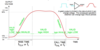4

5

## Show on a graph what the input to output propagation delay for a series of gates is.Where is it measured from?

### When the imput crosses 50% to the output crosses 50%.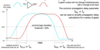6

7

8

## Describe the Thermometer code.Sparse or Dense?Hamming Distance?

### Sparse code.Unit distance.9

## Describe the Gray Code.Sparse or dense?Hamming distance?

### Dense codeUnit-distance circular code10

11

## Describe the BNN (Binary Natural Number) codeRange?Resolution?

### Similar to base-2 notation but 1 is true instead of one and 0 is false not zero.Range: 0 to (2N - 1)Resolution: uniform, unity12

## Describe the SM (Sign Magnitude) number code.Range?Resolution?

### Essentially BNN but an extra bit to multiply result by -1Range: -(2(N-1) - 1) to +(2(N-1) - 1)Resolution: uniform, unity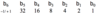13

## Describe the 1'sC (One's complement) number code.Range?Resolution?

### For positive values (first bit is 0) is just BNN For negative values (first bit is 1) then invert remaining bits and take BNN.Range: -(2(N-1) - 1) to +(2(N-1) - 1)Resolution: uniform, unity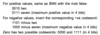14

## Describe the offset BNN codeRange?Resolution?

### Add a positive bias constant to the valu eto be encoded then encode with BNN. To get the value from the codeword subtract the bias from the BNN value of the codeword.Range: Total is 2N, limits set by biasResolution: uniform, unity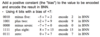15

## Describe the 2'sC (Two's Complement) number code.Resolution?Range?

### Like BNN but most significant bit has negative valueRange: -2(N-1) to 2(N-1) - 1Resolution: uniform, unity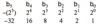16

## Describe the fixed point number code, including what F fractional bits means.Range?Resolution?

### There is a "fraction point" which splits the bits into F fractional bits and N-F other bits. Is usually used with the other codes such as BNN, 2'sC, etc.Range: 0 to approx 2N-F + 1Resolution: uniform, 2-F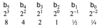17

## Describe the Binary Coded Decimal (BCD) number code.When would you use it over the more conventional codes?

### A code within a code. Split the code into 4-bit fields each of which is used to represnt a single decimal digit 0-9. Thus there are 6 unused codewords (10-15).Useful for legally exact financial calculations, where very little arithmetic processing is needed.18